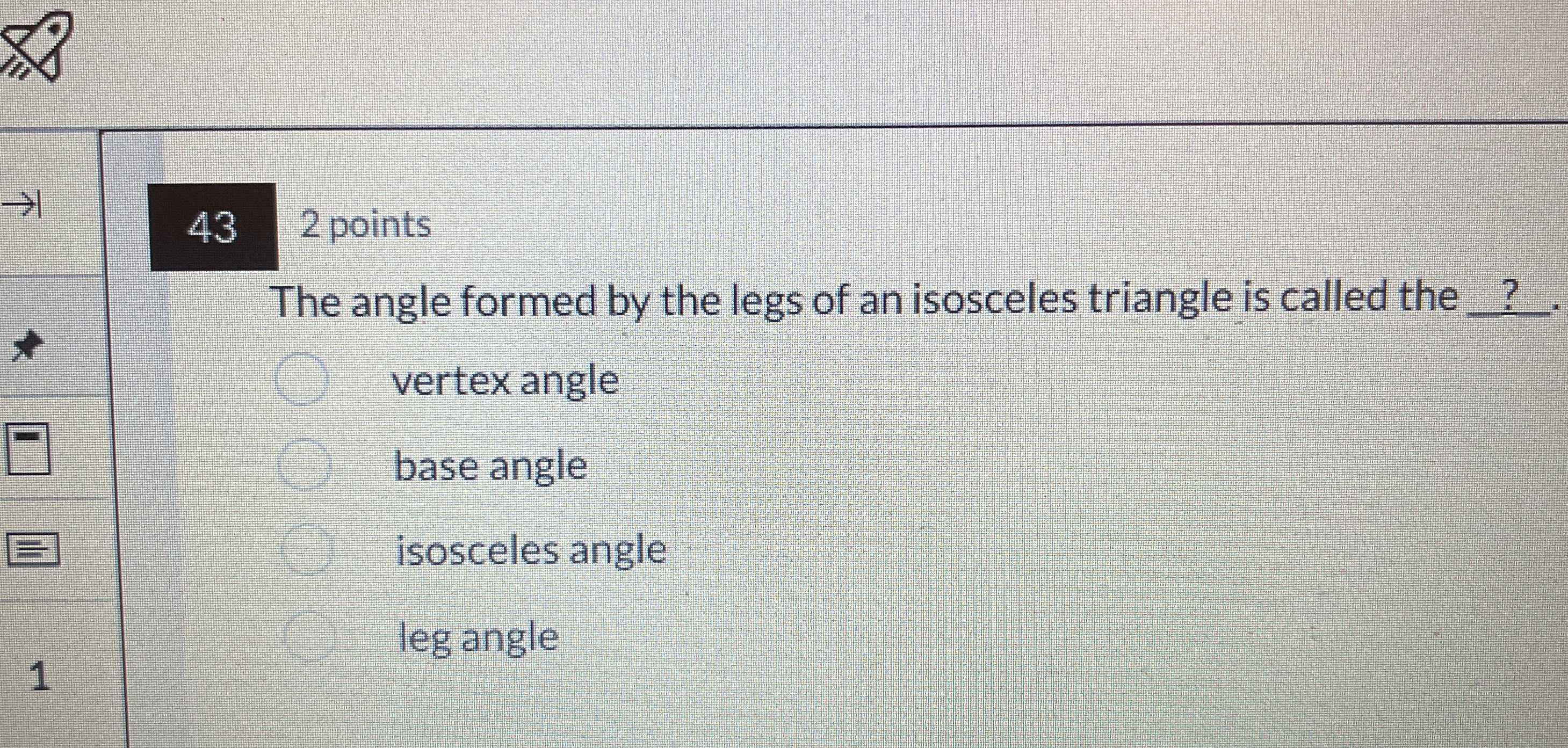### Still have math questions?

Trigonometry
QuestionThe angle formed by the legs of an isosceles triangle is called the $$?$$

A. vertex angle

B. base angle

C. isosceles angle

D. leg angle